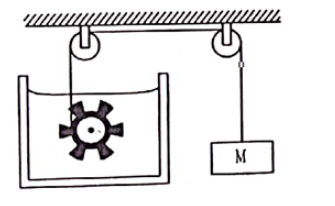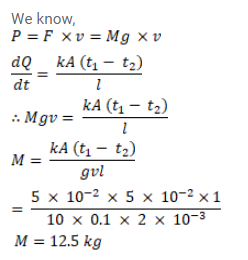# Figure shows water in a container having 2.0 mmQuestion:

Figure shows water in a container having $2.0 \mathrm{~mm}$ thick walls made of a material of thermal conductivity $0.50 \mathrm{~W} / \mathrm{m}-{ }^{\circ} \mathrm{C}$ The container is kept in a melting ice bath at $0^{\circ} \mathrm{C}$ The total surface area in contact with water is $0.05 \mathrm{~m}^{2}$ A wheel is clamped inside the water and is coupled to a block of mass $M$ as shown in the figure. As the block goes down, the wheel rotates. It is found that after some time a steady state is reached in which the block goes down with a constant speed of $10 \mathrm{~cm} / \mathrm{s}$ and the temperature of the water remains constant at $1.0^{\circ} \mathrm{C}$ Find the mass $\mathrm{M}$ of the block. Assume that the heat flows out of the water only through the walls in contact. Take $g=10 \mathrm{~m} / \mathrm{s}^{2}$.Solution: